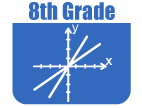Resources by Strand

Strand: MATHEMATICAL PRACTICES (8.MP)
The Standards for Mathematical Practice in Eighth Grade describe mathematical habits of mind that teachers should seek to develop in their students. Students become mathematically proficient in engaging with mathematical content and concepts as they learn, experience, and apply these skills and attitudes (Standards 8.MP.18).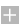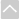Strand: NUMBER SYSTEM (8.NS)
Know that there are numbers that are not rational, and approximate them by rational numbers (Standards 8.NS.1–3).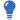Core Guide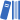OER Curriculum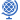Instructional TasksReview Activitiy

Standard 8.NS.1
Know that numbers that are not rational are called irrational. Understand informally that every number has a decimal expansion; for rational numbers show that the decimal expansion repeats eventually, and convert a decimal expansion which repeats eventually into a rational number.

Standard 8.NS.2
Use rational approximations of irrational numbers to compare the size of irrational numbers, locate them approximately on a number line diagram, and estimate the value of expressions (e.g., π2). For example, by truncating the decimal expansion of √2, show that √2 is between 1 and 2, then between 1.4 and 1.5, and explain how to continue on to get better approximations.

Standard 8.NS.3
Understand how to perform operations and simplify radicals with emphasis on square roots.Strand: EXPRESSIONS AND EQUATIONS (8.EE)
Work with radical and integer exponents (Standards 8.EE.1–4).Review ActivitiyOER CurriculumInstructional Tasks

Standard 8.EE.1
Know and apply the properties of integer exponents to generate equivalent numerical expressions. For example, 32 × 3–5 = 3–3 = 1/33 = 1/27.

Standard 8.EE.2
Use square root and cube root symbols to represent solutions to equations of the form x2 = p and x3 = p, where p is a positive rational number. Evaluate square roots of small perfect squares and cube roots of small perfect cubes. Know that √2 is irrational.

Standard 8.EE.3
Use numbers expressed in the form of a single digit times a whole-number power of 10 to estimate very large or very small quantities, and to express how many times as much one is than the other. For example, estimate the population of the United States as 3 times 108 and the population of the world as 7 times 109, and determine that the world population is more than 20 times larger.

Standard 8.EE.4
Perform operations with numbers expressed in scientific notation, including problems where both decimal and scientific notation are used. Use scientific notation and choose units of appropriate size for measurements of very large or very small quantities (e.g., use millimeters per year for seafloor spreading). Interpret scientific notation that has been generated by technology.

Understand the connections between proportional relationships, lines, and linear relationships (Standards 8.EE.5–6).Review ActivitiyOER CurriculumInstructional Tasks

Standard 8.EE.5
Graph proportional relationships, interpreting the unit rate as the slope of the graph. Compare two different proportional relationships represented in different ways. For example, compare a distance-time graph to a distance-time equation to determine which of two moving objects has greater speed.

Standard 8.EE.6
Use similar triangles to explain why the slope m is the same between any two distinct points on a non-vertical line in the coordinate plane; derive the equation y = mx for a line through the origin and the equation y = mx + b for a line intercepting the vertical axis at b.

Analyze and solve linear equations and inequalities and pairs of simultaneous linear equations (Standards 8.EE.7–8)Review ActivitiyOER CurriculumInstructional Tasks

Standard 8.EE.7
Solve linear equations and inequalities in one variable.

1. Give examples of linear equations in one variable with one solution, infinitely many solutions, or no solutions. Show which of these possibilities is the case by successively transforming the given equation into simpler forms, until an equivalent equation of the form x = a, a = a, or a = b results (where a and b are different numbers).
2. Solve single-variable linear equations and inequalities with rational number coefficients, including equations and inequalities whose solutions require expanding expressions using the distributive property and collecting like terms.
3. Solve single-variable absolute value equations.

Standard 8.EE.8
Analyze and solve pairs of simultaneous linear equations.

1. Understand that solutions to a system of two linear equations in two variables correspond to points of intersection of their graphs, because points of intersection satisfy both equations simultaneously.
2. Solve systems of two linear equations in two variables graphically, approximating when solutions are not integers and estimate solutions by graphing the equations. Solve simple cases by inspection. For example, 3x + 2y = 5 and 3x + 2y = 6 have no solution because 3x + 2y cannot simultaneously be 5 and 6.
3. Solve real-world and mathematical problems leading to two linear equations in two variables graphically. For example, given coordinates for two pairs of points, determine whether the line through the first pair of points intersects the line through the second pair.Strand: FUNCTIONS (8.F)
Define, evaluate, and compare functions (Standards 8.F.1–3)Core GuideOER CurriculumInstructional TasksReview Activitiy

Standard 8.F.1
Understand that a function is a rule that assigns to each input exactly one output. The graph of a function is the set of ordered pairs consisting of an input and the corresponding output. (Function notation is not required in grade 8.)

Standard 8.F.2
Compare properties of two functions each represented in a different way (algebraically, graphically, numerically in tables, or by verbal descriptions). For example, given a linear function represented by a table of values and a linear function represented by an algebraic expression, determine which function has the greater rate of change.

Standard 8.F.3
Interpret the equation y = mx + b as defining a linear function, whose graph is a straight line; give examples of functions that are not linear. For example, the function A = s2 giving the area of a square as a function of its side length is not linear because its graph contains the points (1,1), (2,4) and (3,9), which are not on a straight line.

Use functions to model relationships between quantities (Standards 8.F.4–5).Review ActivitiyOER CurriculumInstructional Tasks

Standard 8.F.4
Construct a function to model a linear relationship between two quantities. Determine the rate of change  and initial value of the function from a description of a relationship or from two (x, y) values, including reading these from a table or from a graph. Interpret the rate of change and initial value of a linear function in terms of the situation it models, and in terms of its graph or a table of values.

Standard 8.F.5
Describe qualitatively the functional relationship between two quantities by analyzing a graph (e.g., where the function is increasing or decreasing, linear or nonlinear). Sketch a graph that exhibits the qualitative features of a function that has been described verbally.Strand: GEOMETRY (8.G)
Understand congruence and similarity using physical models, transparencies, or geometry software (Standards 8.G.1–5).Core GuideOER CurriculumInstructional TasksReview Activitiy

Standard 8.G.1
Verify experimentally the properties of rotations, reflections, and translations:

1. Lines are taken to lines, and line segments to line segments of the same length.
2. Angles are taken to angles of the same measure.
3. Parallel lines are taken to parallel lines.

Standard 8.G.2
Understand that a two-dimensional figure is congruent to another if the second can be obtained from the first by a sequence of rotations, reflections, and translations; given two congruent figures, describe a sequence that exhibits the congruence between them.

Standard 8.G.3
Observe that orientation of the plane is preserved in rotations and translations, but not with reflections. Describe the effect of dilations, translations, rotations, and reflections on two-dimensional figures using coordinates.

Standard 8.G.4
Understand that a two-dimensional figure is similar to another if the second can be obtained from the first by a sequence of rotations, reflections, translations, and dilations; given two similar two-dimensional figures, describe a sequence that exhibits the similarity between them.

Standard 8.G.5
Use informal arguments to establish facts about the angle sum and exterior angle of triangles, about the angles created when parallel lines are cut by a transversal, and the angle-angle criterion for similarity of triangles. For example, arrange three copies of the same triangle so that the sum of the three angles appears to form a line, and give an argument in terms of transversals why this is so.

Understand and apply the Pythagorean Theorem and its converse (Standards 8.G.6–8).Core GuideOER CurriculumInstructional TasksReview Activitiy

Standard 8.G.6
Explore and explain a proof of the Pythagorean Theorem and its converse.

Standard 8.G.7
Apply the Pythagorean Theorem to determine unknown side lengths in right triangles in real-world and mathematical problems in two and three dimensions.

Standard 8.G.8
Apply the Pythagorean Theorem to find the distance between two points in a coordinate system.

Solve real-world and mathematical problems involving volume of cylinders, cones, and spheres (Standard 8.G.9).Review ActivitiyOER CurriculumInstructional Tasks

Standard 8.G.9
Know the formulas for the volumes of cones, cylinders, and spheres and use them to solve real-world and mathematical problems.Strand: STATISTICS AND PROBABILITY (8.SP)
Investigate patterns of association in bivariate data (Standards 8.SP.1–4).Core GuideOER CurriculumInstructional TasksReview Activitiy

Standard 8.SP.1
Construct and interpret scatter plots for bivariate measurement data to investigate patterns of association between two quantities. Describe patterns such as clustering, outliers, positive or negative association, linear association, and nonlinear association.

Standard 8.SP.2
Know that straight lines are widely used to model relationships between two quantitative variables. For scatter plots that suggest a linear association, informally fit a straight line, and informally assess the model fit by judging the closeness of the data points to the line.

Standard 8.SP.3
Use the equation of a linear model to solve problems in the context of bivariate measurement data, interpreting the slope and intercept. For example, in a linear model for a biology experiment, interpret a slope of 1.5 cm/hr as meaning that an additional hour of sunlight each day is associated with an additional 1.5 cm in mature plant height. (Calculating equations for a linear model is not expected in grade 8.)

Standard 8.SP.4
Understand that patterns of association can also be seen in bivariate categorical data by displaying frequencies and relative frequencies in a two-way table. Construct and interpret a two-way table summarizing data on two categorical variables collected from the same subjects. Use relative frequencies calculated for rows or columns to describe possible association between the two variables. For example, collect data from students in your class on whether or not they have a curfew on school nights and whether or not they have assigned chores at home. Is there evidence that those who have a curfew also tend to have chores?The Online Core Resource pages are a collaborative project between the Utah State Board of Education and the Utah Education Network. If you would like to recommend a high quality resource, contact Trish French (Elementary) or Lindsey Henderson (Secondary). If you find inaccuracies or broken links contact resources@uen.org.